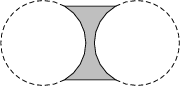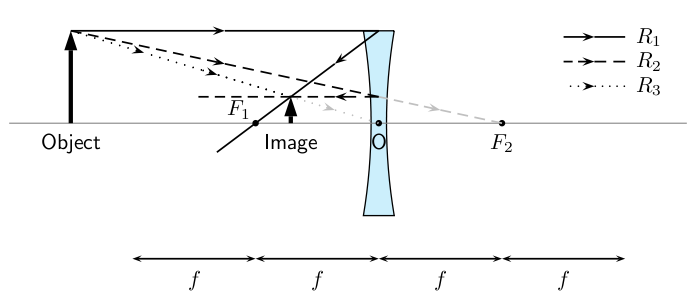# 12.1 Diverging lenses

 Page 1 / 1

## Diverging lenses

We will only discuss double concave diverging lenses as shown in [link] . Concave lenses are thicker on the outside and thinner on the inside.A double concave lens is a diverging lens.

[link] shows a concave lens with light rays travelling through it. You can see that concave lenses have the opposite curvature to convex lenses. This causes light rays passing through a concave lens to diverge or be spread out away from the principal axis. For this reason, concave lenses are called diverging lenses. Images formed by concave lenses are always virtual.Light rays bend away from each other or diverge when they travel through a concave lens. F 1 and F 2 are the foci of the lens.

Unlike converging lenses, the type of images created by a concave lens is not dependent on the position of the object. The image is always upright, smaller than the object, and located closer to the lens than the object.

We examine the properties of the image by drawing ray diagrams. We can find the image by tracing the path of three light rays through the lens. Any two of these rays will show us the location of the image. You can use the third ray to check the location, but it is not necessary to show it on your diagram.

Drawing Ray Diagrams for Diverging Lenses

Draw the three rays starting at the top of the object.

1. Ray ${R}_{1}$ travels parallel to the principal axis. The ray bends and lines up with a focal point. However, the concave lens is a diverging lens, so the ray must line up with the focal point on the same side of the lens where light rays enter it. This means that we must project an imaginary line backwards through that focal point ( ${F}_{1}$ ) (shown by the dashed line extending from ${R}_{1}$ ).
2. Ray ${R}_{2}$ points towards the focal point ${F}_{2}$ on the opposite side of the lens. When it hits the lens, it is bent parallel to the principal axis.
3. Ray ${R}_{3}$ passes through the optical center of the lens. Like for the convex lens, this ray passes through with its direction unchanged.
4. We find the image by locating the point where the rays meet. Since the rays diverge, they will only meet if projected backward to a point on the same side of the lens as the object. This is why concave lenses always have virtual images. (Since the light rays do not actually meet at the image, the image cannot be real.)

[link] shows an object placed at an arbitrary distance from the diverging lens.

We can locate the position of the image by drawing our three rays for a diverging lens.

[link] shows that the image of an object is upright. The image is called a virtual image because it is on the same side of the lens as the object.

The image is smaller than the object and is closer to the lens than the object.Three rays are drawn to locate the image, which is virtual, smaller than the object and upright.

An object is placed 4 cm to the left of a diverging lens which has a focal length of 6 cm.

1. What is the position of the image?
2. Is the image real or virtual?
1. Draw the lens, object, principal axis and focal points.

• ${R}_{1}$ goes from the top of the object parallel to the principal axis. To determine the angle it has when it leaves the lens on the other side, wedraw the dashed line from the focus ${F}_{1}$ through the point where ${R}_{1}$ hits the lens. (Remember: for a diverging lens, the light ray on the opposite side of the lens to the object has to bend away from the principal axis.)
• ${R}_{2}$ goes from the top of the object in the direction of the other focal point ${F}_{2}$ . After it passes through the lens, it travels parallel to the principal axis.
• ${R}_{3}$ goes from the top of the lens, straight through the optical centre with its direction unchanged.
• Just like for converging lenses, the image is found at the position where all the light rays intersect.

2. Draw the image at the point where all three rays intersect.

3. The distance to the object is 2,4 cm.

4. The image is on the same side of the lens as the object, and is upright. Therefore it is virtual. ( Remember: The image from a diverging lens is always virtual.)

## Summary of image properties

The properties of the images formed by converging and diverging lenses depend on the position of the object. The properties are summarised in the [link] .

 Image Properties Lens type Object Position Position Orientation Size Type Converging $>2f$ $<2f$ inverted smaller real Converging $2f$ $2f$ inverted same size real Converging $>f,<2f$ $>2f$ inverted larger real Converging $f$ no image formed Converging $ $>f$ upright larger virtual Diverging any position $ upright smaller virtual

## Diverging lenses

1. An object 3 cm high is at right angles to the principal axis of a concave lens of focal length 15 cm. If the distance from the object to the lens is 30 cm, find the distance of the image from the lens, and its height. Is it real or virtual?
2. The image formed by a concave lens of focal length 10 cm is 7,5 cm from the lens and is 1,5 cm high. Find the distance of the object from the lens, and its height.
3. An object 6 cm high is 10 cm from a concave lens. The image formed is 3 cm high. Find the focal length of the lens and the distance of the image from the lens.

#### Questions & Answers

Can you, if possible send me more quizzes
What is selmon
how long it takes for 25ml ethanol to be evaporated?
how to calculate acceleration
It depends
Mbongeni
Please state the Newton third low
Malwandla
hy
Nelito
Newton's Third law states that to every force applied, there's an equal but opposite reaction
Lala
difference between a head and tail methods
difference between a head-to-tail and tail-to-tail
Zenande
head to tail you draw each vector starting head of the previous vector and tail to tail you construct a parallelogram whereas you started the two vectors from the same axis(from their tails)and the diagonal between the vectors is the resultant vector,tail to tail only includes two vectors
Mbongeni
what is a normal force
a normal force is the force that the surface applies on the object. The force is perpendicular to the surface.
Minaa
Lewis structure for C2H2
what is the mathematical relationship between velocity and time?
How do we find a resulted force of a vector
what is the formula for calculating tension
You can use simultaneous equations or T=mg+ma
sibahle
- Which formula can you use if you want to calculate acceleration?
Pretty
to calculate acceleration you use formula Vf -Vi divided by the time (t)
sholan
What is the formula of calculating tension
I don't think there's a formula u just have to use all information in that type of situation
Gwebzi
can a mixture of atoms make a molecule
yes
Wandile
What is the different between Valence and Valency?
Lil
define the term "resultant"
the sum of all the forces acting on an object
Think
carring out an investigation of the relationship of acceleration and net force with mass kept constant
Ronald
Fnet=ma
Wandile
Hi
Lil
The definition of Newtons Law of Universal Gravitation
Lil
how to calculate coefficient of kinetic force
kinetic friction × Normal force
Murunwa
how to get a valency electronBy Gerr ZenBy RhodesBy